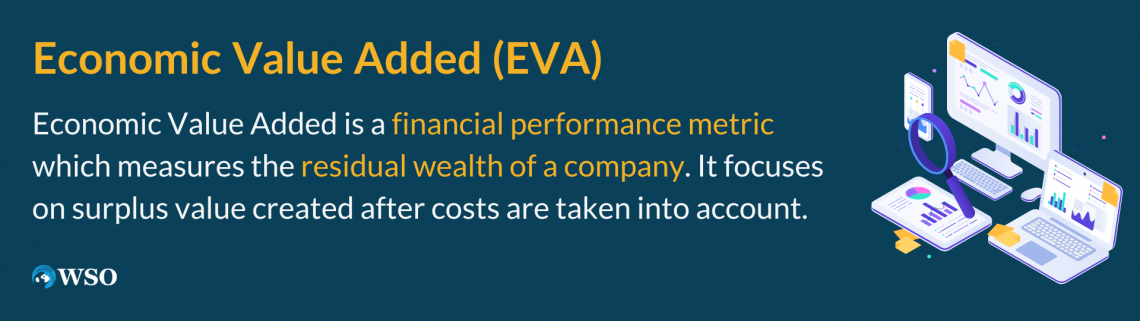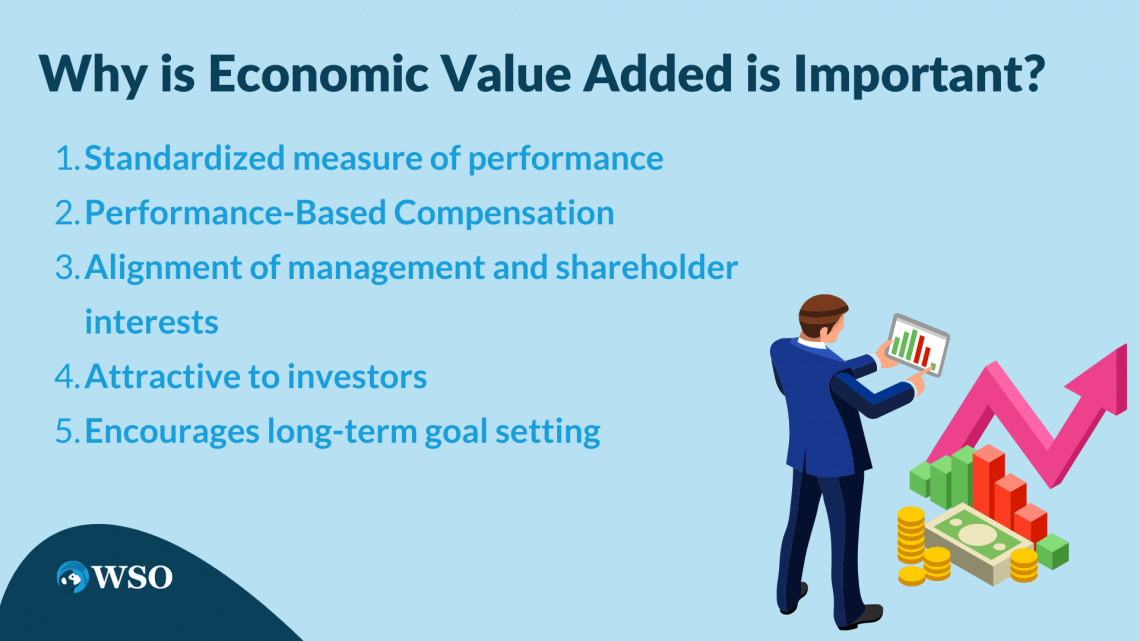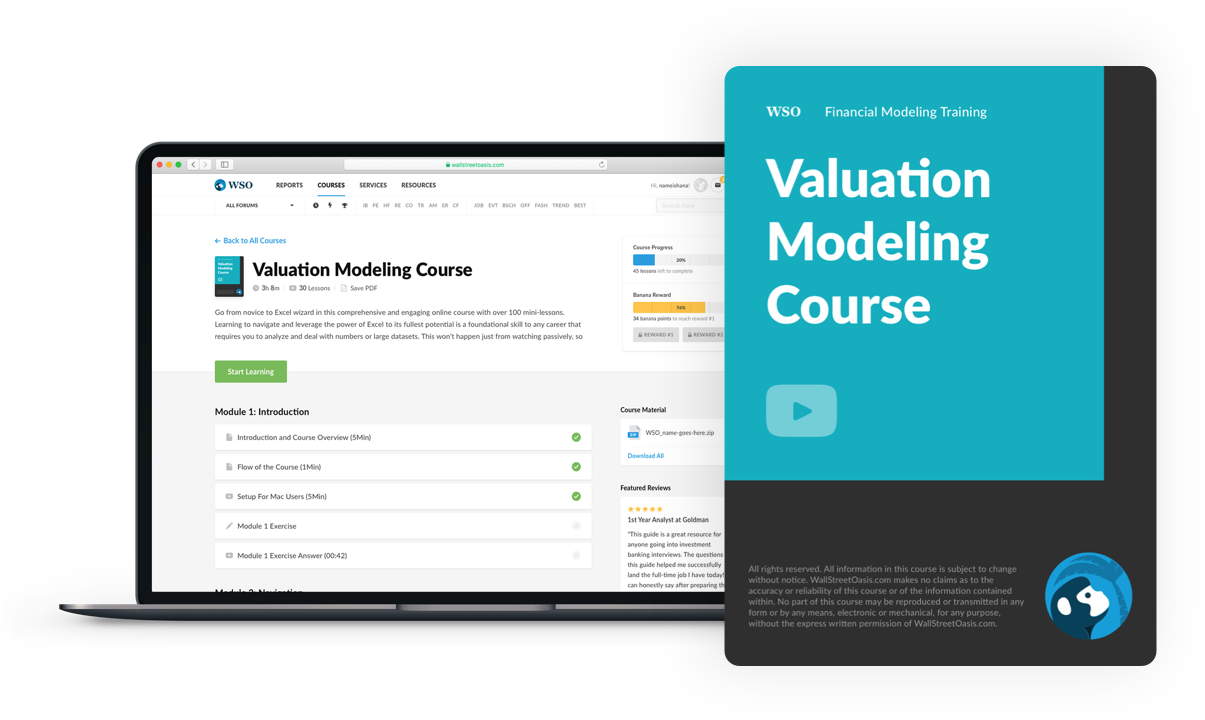# EVA – Economic Value Added

Calculates the difference between a company's net operating profit after taxes and the company's cost of capital

EVA looks at the additional value generated by a company in excess of its cost of capital. Economic Value Added is a financial performance metric measuring a company's residual wealth. It focuses on surplus value created after the costs are considered.This makes it a powerful profitability indicator compared to traditional values such as net income or earnings per share. In addition, by considering the cost of capital, EVA provides a more reliable figure for the true economic value a company generates for its shareholders.

EVA was a metric developed by Stern Stewart and Co., a consulting firm founded in 1982. Joel Stern introduced the concept and Bennett Stewart in their 1991 book "The Quest for Value: EVA Management Guide."

Investors and analysts across various industries often use it to assess the financial health of a corporation. The logic behind this is that real profits are made when an investment project creates returns greater than its capital costs.

## NOTE

EVA computation relies on invested capital and tangible assets, which means the calculation is only useful for mature asset-rich companies.

For example, the information technology sector heavily depends on intangible assets, and EVA cannot capture the value generated by intellectual property.

Today, most companies use it as a reference for strategic decision-making and resource allocation. In addition, it can be used as a cutoff that determines financial compensation and sheds light on balance sheet items that evaluate financial performance.

Overall, there have been major improvements in terms of value creation and company assessment since the introduction of EVA. In addition, it has significantly impacted the financial world by aligning management and shareholder interests more closely.

## EVA Formula & Components Breakdown

The EVA formula calculates a company's additional economic profit above and beyond the cost of capital deployed to generate said profit. The following formula can represent this:

Economic Value Added = NOPAT - (WACC * Invested CapitalWhere;

• NOPAT: Net Operating Profit After Tax
• WACC: Weighted Average Cost of Capital
• Invested Capital: Total amount of capital a company has invested in its core operations

To understand the reasoning behind EVA, it is important to break down its components further. Each element's calculation must be explained before bringing it back together.

1. Net Operating Profit After Tax

The first step to calculate NOPAT requires finding the operating income

Operating Income is simply expressed as Total Revenue - COGS - Operating Expenses. These numbers can be found on a company's income statement

Once the operating income is found, the next step is locating the total taxes paid by a company. Typically, this is seen on the statement of cash flows or income statement under income tax expense.

Now it is easier to calculate the effective tax rate, the total tax expense divided by the operating income previously found. Finally, everything is in place to compute the Net Operating Profit After Tax.

NOPAT = Operating Income * (1 - Effective Tax Rate)2. Weighted Average Cost of Capital

WACC has a more involved process with many facets relating to debt and equity. Its formula is expanded below:

WACC = (Weight of Equity * Cost of Equity) + (Weight of Debt * Cost of Debt)The weights are found by looking at the company's capital structure; total debt and equity figures can be seen on the balance sheet.

Weight of Equity would be Total Equity / (Total Debt + Total Equity) while Weight of Debt is the remaining percentage of Total Debt / (Total Debt + Total Equity).

Moving onto Cost of Equity, this is the expected return on a company's stock. Again, the best way to calculate it is by using the CAPM model, which states the following:

Expected Return = Rf + (Beta * Market Premium)Where;

• Rf: Risk-Free Rate, the rate of return on a risk-free investment such as US 10-y Treasury Bonds
• Beta: measures how volatile a stock is compared to the overall market.
• Market Premium: Market Return minus the risk-free rate, an example of market return is the average return of the S&P 500.

Once the cost of equity is computed, the next course of action is to find the Cost of Debt.
This looks at the interest paid on a company's debt.

It can be described as the interest expense divided by the total debt. These figures can be seen on the income statement and balance sheet. After calculating the cost of debt, all the components are ready in the WACC formula.

3. Invested Capital

There are various ways to calculate invested capital; in this case, debt and equity financing will be used instead of items relating to company assets.

Its formula requires adding total debt and equity and subtracting non-operating assets. Non-operating assets include balance sheet items such as cash, marketable securities, and investments.

The above items have no direct relationship with a company's core business operations. Hence, using non-operating assets gives a clearer picture of the capital a company invested towards its core operations.

Invested Capital = (ST Debt +LT Debt) + Total Equity -Non Operating AssetsFinally, once all these components are known, it is possible to find the economic value added by a company. Subsequently, one can accurately evaluate the true financial performance of a corporation.

## NOTE

EVA is represented as a dollar surplus value, not the percentage difference in excess value for a company.

## Economic Value Added Calculation Example

To demonstrate how the EVA for a company can be found, a 10-K annual report for Chevron (CVX) is used. This provides a practical illustration of the formula discussed previously. (all values are in millions of dollars)Firstly, the Net Operating Profit After Tax for Chevron must be calculated. A snapshot of Chevron's income statement is provided below to help with the NOPAT formula:

Operating Income is total revenue minus COGS and operating expenses. The total revenue for 2021 is $155,606. Operating expenses are set at$20,726.

COGS include any direct costs that go into producing a company's product. For example, this would include purchased crude oil and products ($89,372), SG&A expenses ($20,726), and exploration expenses (549). In total, COGS for 2021 comes to $110,647. Therefore, the operating income will be 155,606 - (110,647 + 20,726) =$24,233. Now that the operating income is known, one can find the income tax expense for Chevron, which is also on the income statement ($5,950). The effective tax rate will subsequently be 24.6% (tax expense of 5,950 / operating income of 24,233). Everything is set for the NOPAT calculation. NOPAT =$24,233 * (1 - 0.246)

NOPAT = $18,283 The 2021 Net Operating Profit After Tax for Chevron is$18,283. The first component of the EVA calculation has been found. Moving on, it is time to calculate the WACC for Chevron.

While WACC estimates are available on financial data sites such as Bloomberg and FactSet, it is better to calculate them manually and cross-check the values. The calculation does not need to be exact, but if a major discrepancy exists, one should go through the inputs used again.

Chevron's balance sheet is given below to help find different WACC components.

The total equity for Chevron is given as $139,940 on its balance sheet, and its total debt can be found as$31,369 (ST debt of 256 + LT debt of 31,113). Equity and debt weights for Chevron can now be computed.

Chevron Weight of Equity = 139,940 / (139,940 + 31,369)
Weight of Equity = 0.817 or 81.7%

Chevron Weight of Debt = 31,369 / (139,940 + 31,369)
Weight of Debt = 0.183 or 18.3%

Next, it is time to compute the cost of debt. This requires Chevron's interest expense which is 712 (located on the income statement under interest and debt expense). The cost of debt can be calculated since total debt is also known.

Chevron Cost of Debt = 712 / 31,369
Cost of Debt = 0.023

The last step is to find Chevron's cost of equity using the CAPM model. To begin, the risk-free rate for June 2021 (1.51%) is taken. This can be converted to a monthly value by doing the following:

Monthly Risk Free Rate = (1 + 0.0151)^(1/12) - 1
Monthly Risk Free Rate = 0.125%

The market return must also be monthly to maintain consistency. For example, according to Yahoo Finance, the S&P 500's monthly return for June 2021 was 2.28%, and Chevron's 5-year monthly Beta is 1.16.

The market premium can now be computed by doing 2.28% minus 0.125% to give a value of 2.16%. This encapsulates all the values needed to calculate the cost of equity.

Chevron Cost of Equity = 0.125% +(1.16 * 2.16%)
Cost of Equity = 0.026

Finally, the 2021 WACC for Chevron can be found using the numbers mentioned above.

Chevron 2021 WACC = (0.817 * 0.026) + (0.183 * 0.023)
WACC = 0.025 or 2.5%

Invested Capital is now the only component of EVA left to calculate. It includes total debt and total equity, which have already been found as $31,369 and$139,940, respectively.

Non-operating assets can be aggregated using balance sheet items such as cash ($5,640), marketable securities ($35), and investments ($40,696). Hence, Chevron's non-operating assets for 2021 are set at$46,371.

Chevron 2021 Invested Capital = (31,369 + 139,940) - 46,371
Invested Capital = $124,938 To conclude, all EVA components have been found with NOPAT being$18,283, a WACC of 2.5% and invested capital set at $124,938. Now calculating economic value added for Chevron in 2021 is straightforward. Chevron 2021 EVA = 18,283 - (2.5% * 124,938) EVA =$15,173

Chevron's EVA was recorded to be $15,173 in 2021. In this calendar year, the company generated a net profit of roughly$15,173 (in millions) after considering the cost of capital.

Chevron has created substantial value for its shareholders and is efficiently using its resources, this provides management with a clear idea regarding financial performance, and they can either decide to keep operations as they are or focus on reducing capital costs further.

## Why is EVA important?

Calculating EVA is tedious, and it is crucial to understand why investors and analysts treat it as one of the most important performance metrics in finance.There are several reasons why EVA or economic value added has made a significant impact since its introduction in the 1990s:

1. Standardized measure of performance

Previously, it was difficult to compare companies in different industries. Still, since the introduction of EVA, it is possible to track which corporations are creating the most economic value across sectors.

2. Performance-Based Compensation

It is a metric that can be looked at when deciding compensation for executives. EVA is an unbiased measure that incentivizes value creation because employees will get fairly rewarded for doing so.

3. Alignment of management and shareholder interests

The overarching aim of corporations has been to create shareholder value since Milton Friedman's 1970 article stated that managers must conduct business according to shareholder desires.

## NOTE

EVA is a performance indicator that directly works towards this goal. By identifying the economic value added, a company can accurately measure the extent to which shareholder needs are met.

4. Attractive to investors

A positive EVA will attract investors as they are constantly looking for companies that generate profits beyond their cost of capital.

This would create a self-enforcing cycle where stock price moves up due to investor demand, and capital costs are further reduced. Hence, the company's EVA would improve, attracting even more investors.

5. Encourages long-term goal setting

If a company focuses on EVA as a performance metric, it encourages management to make decisions that create value in the long haul.

This would create a more robust strategy, shifting targets from short-term gains to value growth that can sustain itself over larger periods.

On a micro level, it can be used across multiple divisions in a company to discern high-growth areas and allocate resources accordingly. From a macro standpoint, EVA allows for a standardized comparison of value created for companies in contrasting industries.

In conclusion, Economic Value Added is a measure that has greatly impacted business strategy by assessing whether a company is creating excess value on its investments.

## Key Takeaways

• EVA measures financial performance by looking at the additional value created after considering capital costs.
• It helps quantify the financial health of a company. This allows management to create informed strategies for sustainable growth and efficient resource allocation.
• The Economic Value Added formula focuses on NOPAT, WACC, and invested capital. These components can be further broken down by finding the relevant line items on the balance sheet and income statement.
• Invested Capital is a major part of the formula and does not consider the influence of patents, trademarks, and brand reputation. Hence, EVA might not accurately reflect the value created by companies that rely on intangible assets.
• Investors and analysts place a lot of importance on this performance indicator because EVA is a standardized measure that caters to shareholder interests.
• Companies strive to have a positive EVA as it will attract investors, boost share price and provide greater compensation figures for executives.### Everything You Need To Master Valuation Modeling

To Help You Thrive in the Most Prestigious Jobs on Wall Street.

Researched and Authored by Amrit Kachroo | LinkedIn
Reviewed and edited by Parul Gupta | LinkedIn